# NCERT Solutions for Class 8 Maths Exercise 1.2## myCBSEguide App

CBSE, NCERT, JEE Main, NEET-UG, NDA, Exam Papers, Question Bank, NCERT Solutions, Exemplars, Revision Notes, Free Videos, MCQ Tests & more.

NCERT Solutions for Class 8 Maths Exercise 1.2 Class 8 Maths book solutions are available in PDF format for free download. These ncert book chapter wise questions and answers are very helpful for CBSE exam. CBSE recommends NCERT books and most of the questions in CBSE exam are asked from NCERT text books. Class 8 Maths chapter wise NCERT solution for Maths Book all the chapters can be downloaded from our website and myCBSEguide mobile app for free.

NCERT solutions for Class 8 Maths Rational Numbers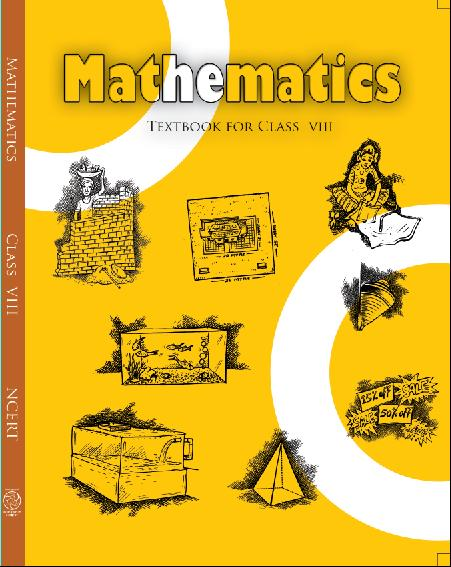## NCERT Solutions for Class 8 Maths Rational Numbers

###### 1. Represent these numbers on the number line:

(i)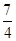(ii)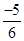Ans 1. (i)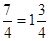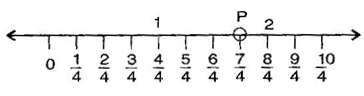Here, P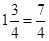(ii)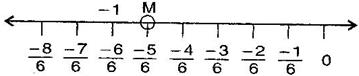Here, M =NCERT Solutions for Class 8 Maths Exercise 1.2

###### 2. Represent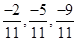on the number line.

Ans 2. Here, B =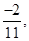C =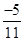and D =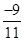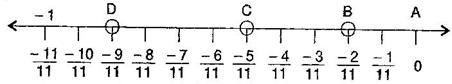NCERT Solutions for Class 8 Maths Exercise 1.2

###### 3. Write five rational numbers which are smaller than 2.

Ans 3.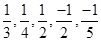and so on.

###### 4. Find ten rational numbers between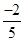and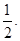Ans 4.and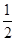Here, L.C.M. of 5 and 2 is 10.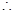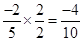and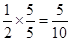Again,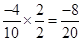and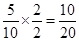Ten rational number betweenandare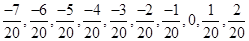.

NCERT Solutions for Class 8 Maths Exercise 1.2

###### 5. Find five rational numbers between:

(i)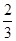and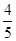(ii)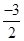and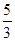(iii)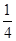andAns 5. (i)andL.C.M. of 3 and 5 is 15.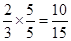and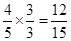Again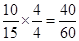and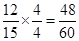Five rational numbers betweenandare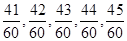.

(ii)andL.C.M. of 2 and 3 is 6.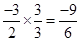and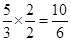Five rational numbers betweenandare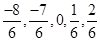.

(iii)andL.C.M. of 4 and 2 is 4.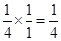and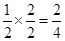Again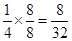and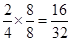Five rational numbers betweenandare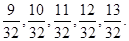NCERT Solutions for Class 8 Maths Exercise 1.2

###### 6. Write 5 rational numbers greater than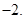Ans 6. Five rational numbers greater than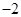are: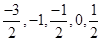[Other rational numbers may also be possible]

NCERT Solutions for Class 8 Maths Exercise 1.2

###### 7. Find ten rational numbers between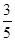and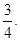Ans 7.and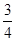L.C.M. of 5 and 4 is 20.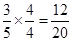and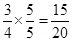Again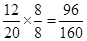and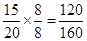Five rational numbers betweenandare: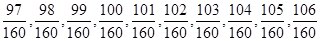.

## NCERT Solutions for Class 8 Maths Exercise 1.2

NCERT Solutions Class 8 Mathematics PDF (Download) Free from myCBSEguide app and myCBSEguide website. Ncert solution class 8 Mathematics includes text book solutions from Class 8 Maths Book . NCERT Solutions for CBSE Class 8 Maths have total 16 chapters. 8 Maths NCERT Solutions in PDF for free Download on our website. Ncert class 8 solutions PDF and Maths ncert class 8 PDF solutions with latest modifications and as per the latest CBSE syllabus are only available in myCBSEguide.

## CBSE app for Class 8

To download NCERT Solutions for class 8 Social Science, Computer Science, Home Science,Hindi ,English, Maths Science do check myCBSEguide app or website. myCBSEguide provides sample papers with solution, test papers for chapter-wise practice, NCERT solutions, NCERT Exemplar solutions, quick revision notes for ready reference, CBSE guess papers and CBSE important question papers. Sample Paper all are made available through the best app for CBSE students and myCBSEguide website.### 16 thoughts on “NCERT Solutions for Class 8 Maths Exercise 1.2”

1. It’s very helpful for me

2. In questions 5 part 1
In second line,why we multiple the number with 4

3. It is very helpful to me and my sister and my friends

4. Very good method of your teaching I like your sum

5. Thanks for it

6. It’s very helpful for me

7. Very very much thankful to you

8. It’s very helpful to our generation .

9. how can we solve the ques no 6 of ex 1.2

10. Solve completely

11. It is very helpful for class 8th studends

12. Thank u so much.
My madam is very strickt and she want to do all homework .
U r great????????

13. thank u so much its very useful

14. This is very helpful for me thanks

15. Thnx it is verry helpfull

16. Ishika it is very useful to generation